Q1. Determine the minimum high input voltage(+V) required to saturate the transistor switch shown in Fig. 1.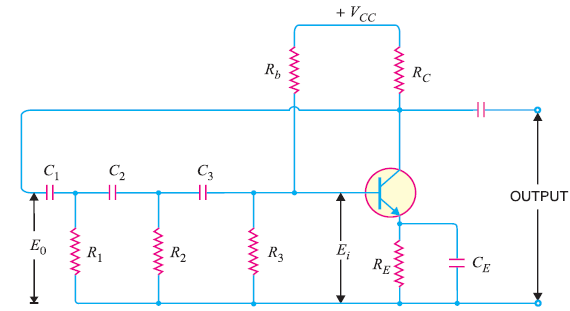Fig.1

Solution.

Assuming the transistor to be ideal,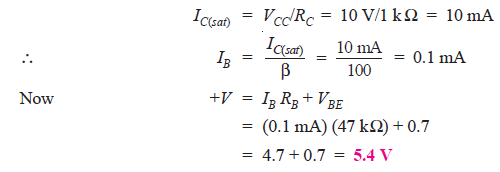Hence in order to saturate the transistor, we require + 5.4 V.

Q2. A transistor is used as a switch. If VCC = 10V, RC = 1 kΩ and ICBO = 10 μA, determine the value of VCE when the transistor is (i) cut off and (ii) saturated.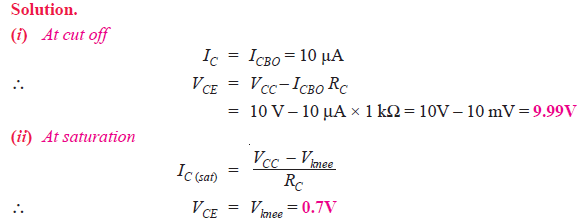Q3. Fig. 2 shows the transistor switching circuit. Given that RB = 2.7 kΩ, VBB = 2V, VBE = 0.7V and Vknee = 0.7V.
(i) Calculate the minimum value of β for saturation.
(ii) If VBB is changed to 1V and transistor has minimum β = 50, will the transistor be saturated.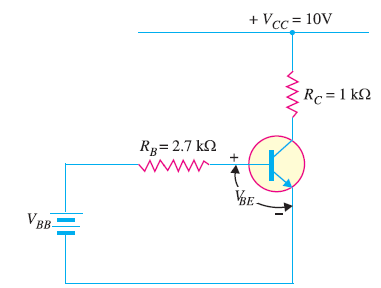Fig.2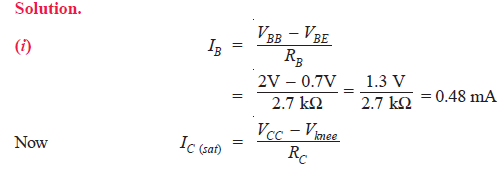Since the collector current is less than saturation current (= 9.3 mA), the transistor will not be saturated.

Q4.In the astable multivibrator shown in Fig. 3, R2 = R3 = 10 kΩ and C1 = C2 = 0.01 μF. Determine the time period and frequency of the square wave.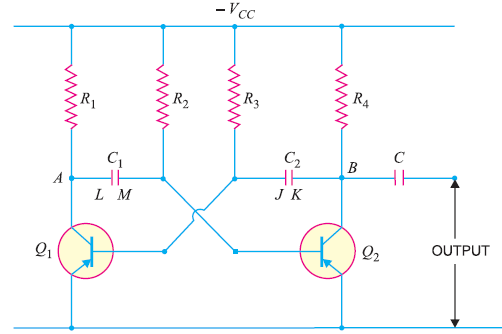Fig.3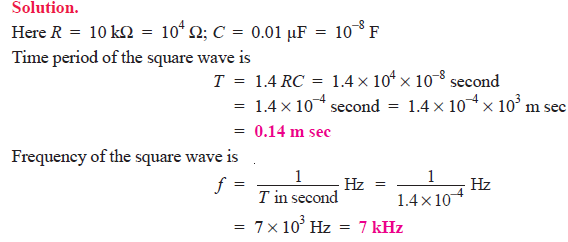Q5. In a differentiating circuit, R = 10 kΩ and C = 2.2 μF. If the input voltage goes from 0 V to 10 V at a constant rate in 0.4 s, determine the output voltage.

Solution.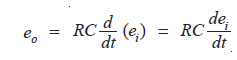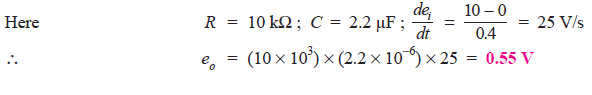Q6. For the negative series clipper shown in Fig.4, what is the peak output voltage from the circuit?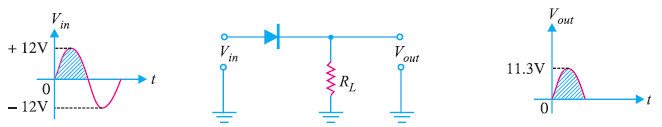Fig.4

When the diode is connected in series with the load, it is called a series clipper. Since it is a negative clipper, it will remove a negative portion of input a.c. signal.

During the positive half-cycle of input signal, the dioide is forward biased. As a result, the diode will conduct. The output voltage is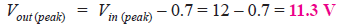During the negative half-cycle of input signal, the diode is reverse biased and consequently, it will not conduct. Therefore, Vout = 0. Note that under this condition, the entire input voltage will appear across the diode.

Q7. The negative shunt clipper shown in Fig. 5 (i) has a peak input voltage of + 10 V. What is the peak output voltage from this circuit ?Fig.5

Solution.

When the diode is connected in parallel with the load, it is called a shunt clipper. During the positive half-cycle of input ac signal, the diode is reverse biased and it will behave as an open. This is shown in Fig. 5 (ii). With diode as an open,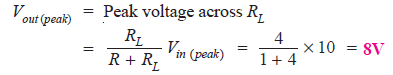Note that peak output voltage is somewhat less than the peak input voltage.

Q8. The positive shunt clipper shown in Fig. 6 has the input waveform as indicated. Determine the value of Vout for each of the input alternations.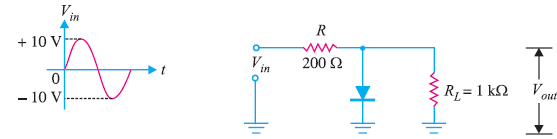Fig.6

Solution.
Positive half-cycle: During the positive half-cycle of the input ac signal, the diode is forward biased. Therefore, diode can be replaced by its simplified equivalent circuit as shown in Fig. 7. Since the load is connected in parallel with the diode,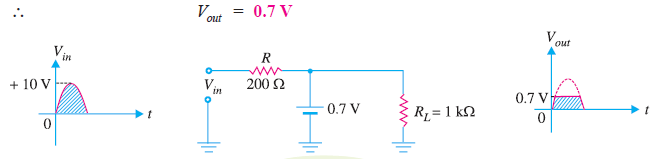Fig.7

Negative half-cycle: During the negative half-cycle of the input a.c. signal, the diode is reverse biased and it conducts no current. Therefore, the diode will behave as an open as shown in Fig. 8.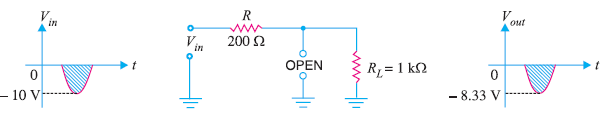Fig.8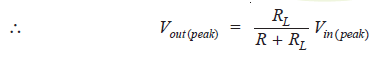Again the peak output voltage is somewhat less than the peak input voltage.

Q9. For the input wave to the clipping circuit shown in Fig. 9, find the output waveform.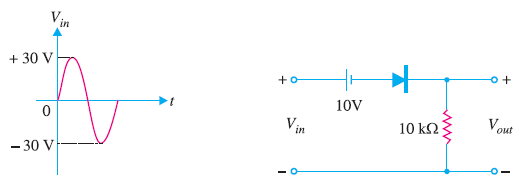Fig.9

Solution. For any value of Vin > 10V, the ideal diode is forward biased and Vout = Vin – 10. For example, at Vin = 15V [See Fig. 10 (i)], Vout = 15 – 10 = 5V.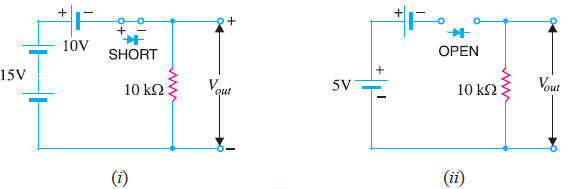Fig.10

For any value of Vin < 10V, the ideal diode is reverse biased. Therefore, circuit current is zero and hence Vout = 0. For example, with Vin = 5V [See Fig.10 (ii)], Vout = 0 and Vd (drop across diode) = 5V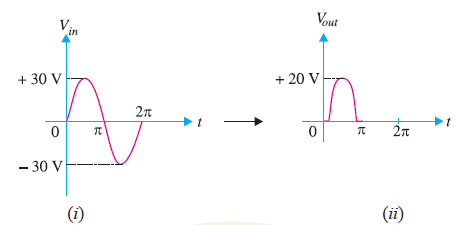Fig.11

The output waveform will be as shown in Fig. 11 (ii).

Q10. For the input wave to the clipping circuit in Fig. 12, find the output waveform.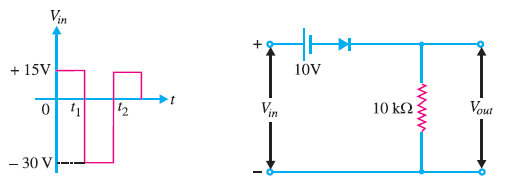Fig.12

Solution. For any value of Vin > 10V, the ideal diode is forward biased and Vout = Vin – 10. For any value of Vin < 10V, the ideal diode is reverse biased and Vout = 0.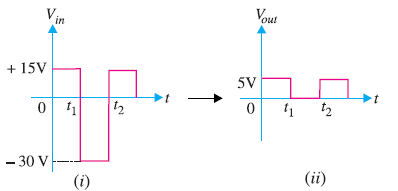Fig.13

The output waveform will be as shown in Fig. 13 (ii).

Q11. For the input wave to the symmetrical clipper shown in Fig. 14, find the output voltage waveform. Assume that the barrier voltage of each diode is 0.6V.

Solution. Fig. 14 shows the symmetrical clipper.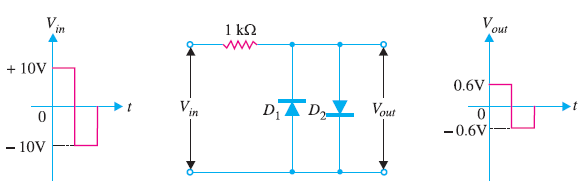Fig.14

(i) Diode D1 is reverse biased for all positive inputs and negative inputs less than 0.6V. Diode D2 is reverse biased for all negative inputs and positive inputs less than 0.6V.
(ii) For all positive inputs greater than 0.6V, D2 is forward biased and output voltage stays at 0.6V. For all negative inputs greater than –0.6V, D1 is forward biased and output stays at – 0.6V.

Thus for the input voltage waveform, the output voltage varies between – 0.6V and 0.6V. This circuit is sometimes used to convert a sine-wave input to a reasonably square-wave output signal.

Q12. Fig. 15 shows a zener shunt clipper with sine wave input. Determine the output waveform.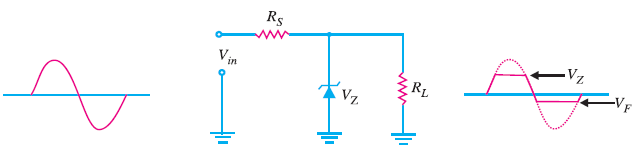Fig.15

Solution.

The Zener shunt clipper uses a Zener diode in place of the ordinary diode. The Zener shunt clipper uses both the forward and reverse characteristics of the Zener diode. Thus when the Zener diode is forward biased, this clipper acts just like the standard shunt clipper.
When the input signal in Fig. 15, goes positive, the Zener is reverse biased. Therefore, the Zener diode will remain OFF until the value of Vin reaches the value of VZ. At that time, the Zener diode will turn ON, clipping the input signal. As long as Vin is greater than VZ, the Zener will remain ON and the output voltage is

Vout = VZ

When the input signal goes negative, the Zener is forward biased. Therefore, the Zener is clipped off at 0.7 V.

Q13. Fig. 16 shows a symmetrical Zener shunt clipper with sine wave input. Determine the output waveform.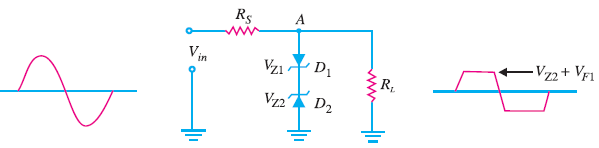Fig. 16

Solution.

The symmetrical zener clipper uses two zener diodes that are connected as shown in Fig. 16.

When the input is positive, D1 is forward biased and D2 is reverse biased (assuming that the value of Vin is high enough to turn both diodes ON). When both diodes are conducting, the voltage from point A to the ground will be equal to the sum of VZ2 and the forward voltage drop of D1 i.e., VF1 = 0.7V.When the input is negative, D1 is reverse biased and D2 is forward biased. The output voltage in this case is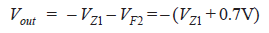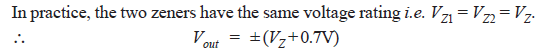Q14. Sketch the output waveform for the circuit shown in Fig. 17. It is given that discharging time constant (CRL) is much greater than the time period of the input wave.Fig.17

Solution.
During positive half-cycle of the input signal, the diode is forward biased. The network will appear as shown in Fig. 18. It is clear that Vout = + 2 V.

Further, applying Kirchhoff’s voltage law to the input loop in Fig. 18, we have,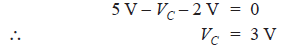Therefore, the capacitor will charge up to 3 V.

During the negative half-cycle of the input signal, the diode is reverse biased and will behave as an open [See Fig. 19]. Now battery of 2 V has no effect on Vout.

Applying Kirchhoff’s voltage law to the outside loop of Fig. 19, we have,The negative sign results from the fact that the polarity of 8 V is opposite to the polarity defined for Vout. The clamped output is shown in Fig. 20. Note that the output swing of 10 V matches with the input swing.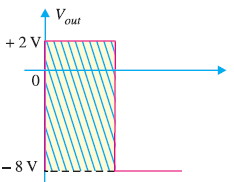Fig.20